##### Quantum Physics For Dummies, Revised EditionIn quantum physics, to be able to determine the energy levels of a particle in a box potential, you need an exact value for X(x) — not just one of the terms of the constants A and B. You have to use the boundary conditions to find A and B. What are the boundary conditions? The wave function must disappear at the boundaries of the box, so

• X(0) = 0

• X(Lx) = 0

So the fact that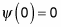tells you right away that B must be 0, because cos(0) = 1. And the fact that X(Lx) = 0 tells you that X(Lx) = A sin(kxLx) = 0. Because the sine is 0 when its argument is a multiple of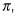this means that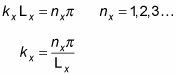And because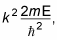it means that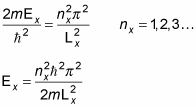That's the energy in the x component of the wave function, corresponding to the quantum numbers 1, 2, 3, and so on. The total energy of a particle of mass m inside the box potential is E = Ex + Ey + Ez. Following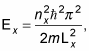you have this for Ey and Ez: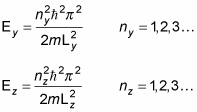So the total energy of the particle is E = Ex + Ey + Ez, which equals this: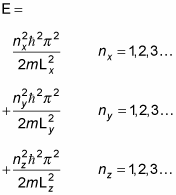And there you have the total energy of a particle in the box potential.